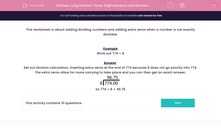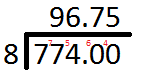# Short Division: Three-Digit Numbers and Remainders

In this worksheet, students will practise division of three-digit numbers by a number that does not divide exactly.Key stage:  KS 2

Curriculum topic:   Maths and Numerical Reasoning

Curriculum subtopic:   Mixed Problems

Difficulty level:#### Worksheet Overview

This activity is about dividing numbers and adding extra zeros when a number is not exactly divisible.

Example

Work out 774 ÷ 8

Set out your division calculation, inserting extra zeros at the end of 774 because 8 does not go exactly into 774. The extra zeros allow for more carrying to take place and you can then get an exact answer.So 774 ÷ 8 = 96.75

Let's get started!

### What is EdPlace?

We're your National Curriculum aligned online education content provider helping each child succeed in English, maths and science from year 1 to GCSE. With an EdPlace account you’ll be able to track and measure progress, helping each child achieve their best. We build confidence and attainment by personalising each child’s learning at a level that suits them.

Get started